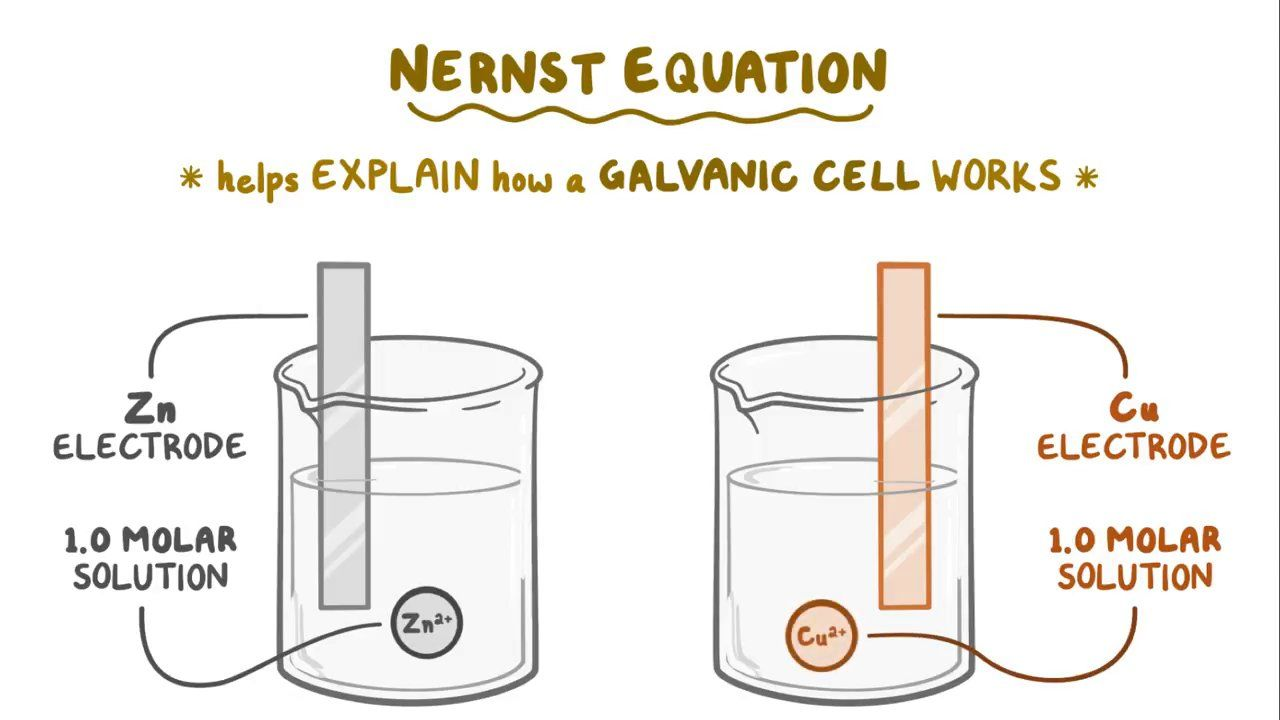[Image_Link]https://www.facebook.com/tr?id=824012811003867&ev=PageView&noscript=1 />

# Nernst equation

874views00:00 / 00:00

## Nernst equation

Cellular biology

### Cellular biology

Cellular structure and function

Cell membrane

Selective permeability of the cell membrane

Extracellular matrix

Cell-cell junctions

Endocytosis and exocytosis

Osmosis

Resting membrane potential

Nernst equation

Cytoskeleton and intracellular motility

Cell signaling pathways

### Disorders of cellular biology

Leukodystrophy

Zellweger spectrum disorders (NORD)

Primary ciliary dyskinesia

Alport syndrome

Ehlers-Danlos syndrome

Osteogenesis imperfecta

Marfan syndrome

Vitamin C deficiency

Peroxisomal disorders: Pathology review

Nernst equation

0 / 13 complete

of complete

### Transcript

#### Content Reviewers

Rishi Desai, MD, MPH

#### Contributors

William Gilpin

Evan Debevec-McKenney

Justin Ling, MD, MS

The Nernst equation helps explain how a Galvanic cell works - so let’s start by drawing out a galvanic cell.

A galvanic cell has two electrodes - so for our example, let’s say there’s one solid zinc electrode and that it’s in a 1.0 molar solution of zinc-two-plus ions, and that there’s a solid copper electrode and it’s in a 1.0 molar solution of copper-two-plus ions.

Now, copper ions turn their solution a blue color, whereas zinc ions don’t color their solution - so it’s colorless.

Since the concentration of both copper and zinc ion solutions is 1.0 molar and if we assume that the temperature is 25 degrees celsius, then we’re under standard conditions.

Now, in our galvanic cell, the two electrodes are connected by a wire with an open switch and there’s also a salt bridge between the two compartments.

The salt bridge allows electrical charge to travel between the two solutions.

To get the galvanic cell started, we need to close the switch, so that electrons or electrical current can flow through the wire.

Now, if we want to calculate the cell potential, or cell voltage, for this zinc-copper cell, we need to use the Nernst equation.

So here’s the Nernst equation, E refers to the instantaneous voltage of the galvanic cell, or the voltage at a specific moment in time.

Enaught or Ezero is a constant called the “standard voltage,” and it’s simply based on the two metals that we’re using in our galvanic cell.

We can look up this number for zinc and copper, and we find that it has a value of +1.10 volts.

N refers to the number of moles of electrons that are transferred during the redox reaction.

Q is the reaction quotient, which is the ratio of the concentration of reactants to the concentration of products in our galvanic cell.

When we calculate this, we only include the concentration of the ions, and we leave out the pure solids like the zinc and copper electrodes. This tells us the progress of the reaction.

### Summary

The Nernst equation is a mathematical relationship that explains how a Galvanic cell works and allows us to find the instantaneous voltage of a cell at a specific moment in time.

Its expression is:

E = E0 - (RT/zF)lnQ

Where:

E is the potential difference across the membrane (in volts) E0 is the equilibrium potential for the ion (in volts) R is the universal gas constant T is the temperature (in Kelvin) z is the charge of the ion F is the Faraday constant Q is the reaction quotient